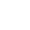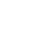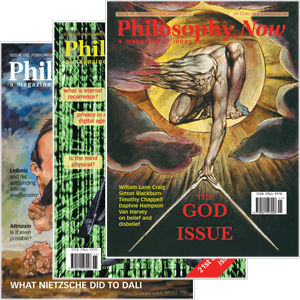# Mathematical Knowledge: A Dilemma

### Rui Vieira thinks of a number.

What exactly are the objects of mathematics, and how do they relate to our knowledge of them? Since Plato (427 BC–347 BC) such questions have been central to the philosophy of mathematics. Plato realized that mathematics seems to involve perfect circles, triangles, and so on. But as Plato also noticed, there are no perfect circles or triangles to be found in the world, only imperfect approximations. Imagine a polygon [shape] with an ever-increasing number of equal sides. As the number of sides approaches infinity, the polygon will become a circle. Thus a perfect circle may be conceived of as a regular polygon with an infinite number of infinitesimally small sides. So no matter how accurate our or our computer’s rendering of a circle may be, it will only be an imperfect approximation. Finite humans and their computers cannot create objects with infinite mathematical features, such as the infinite sides of our ideal circle. Plato concluded that since there are no perfect mathematical objects to be found in the world, the objects of mathematics – perfect circles, triangles, and indeed numbers themselves – must somehow exist as eternal abstract entities beyond space and time in some otherworldly Platonic heaven called the world of Forms (or Ideas). Plato’s particular type of mathematical realism (ie, of attributing objective reality to mathematical objects), has been one of the most prevalent views of mathematics among both philosophers and mathematicians ever since.

But for many philosophers after Plato – particularly the empiricists, who view all knowledge as ultimately acquired through experience – this transcendental view of mathematics was implausible. Thus, since Plato’s time, the history of the philosophy of mathematics has also been replete with anti-Platonist accounts attempting to bring mathematics back down to earth from its heavenly perch. One such early Twentieth Century account, called conventionalism, viewed mathematical statements as ‘analytic’ or true by convention. An analytic statement is any statement that’s true solely because of the conventional meaning of its terms – such as “All bachelors are unmarried”. Similarly, mathematical statements were to be viewed as true solely because of the conventional definition of their terms. Also in the early Twentieth Century, mathematician David Hilbert was pre-eminent in developing formalism, the view that mathematics is nothing more than the rule-based manipulation of symbols whose meanings are largely unimportant. Around the same time, mathematician L.E.J. Brouwer developed intuitionism, which viewed mathematical statements as being constructed through our subjective intuitions. These accounts of mathematics all rejected Platonism by avoiding any reference to mind-external mysterious abstract mathematical objects.

However, in an important paper, ‘Mathematical Truth’, in the Journal of Philosophy, Vol. 70 (1973), philosopher Paul Benacerraf argued that anti-Platonist accounts of mathematics deprive mathematical statements of their objective truth in the everyday popular sense, ie of the idea that mathematical truths are true whether anyone thinks about them or not. Objective truth is a property of mathematics which for most of us is obvious, but anti-Platonist accounts render mathematics subjective (although Benacerraf’s argument is directed at conventionalism and formalism, I don’t think intuitionism fares any better).

In his paper, Benacerraf shows that mathematical statements such as “There are at least three perfect numbers greater than 17” have the same grammatical and logical form as non-mathematical statements such as “There are at least three large cities older than New York”. Thus, Benacerraf contends that if mathematical statements are to be true in the same objective way that statements are true of everyday objects or facts, then the objects of mathematical statements must similarly exist independent of our subjective conventions or forms. That is, just as true statements about real cities must be true of objectively-existing cities, so must our true mathematical statements about numbers, perfect circles and the like, be true of objectively-existing numbers, perfect circles and the like. It would seem, therefore, that Platonism is the only mathematical account which would allow for the objective truth of mathematics.

## How Do We Know Mathematical Truths?

However, a problem apparently besetting Platonism is also posed by Benacerraf. If mathematical objects such as numbers, perfect circles exist as abstract entities beyond space and time, neither affected by nor causing anything in our everyday world, as Platonism asserts, then how is it that we can come to have knowledge of them? If we must posit the existence of Platonic mathematical objects to make mathematical statements objectively true, then we are left with a dilemma: either to say that mathematics is subjective, or to explain how we ever have knowledge of inaccessible non-spatiotemporal objects.

I think only particularly anti-Platonist and whollyempiricist accounts of mathematics can fruitfully respond to this epistemological dilemma. In his influential book The Nature of Mathematical Knowledge (1984), philosopher Philip Kitcher proposed just such an account by updating the mathematical empiricism of John Stuart Mill. For Kitcher, mathematics consists not of abstract Platonic entities, but of generalized human empirical operations performed on physical objects: such as the collecting, correlating or segregating of, for example, pebbles. For instance, the mathematical statement 2 + 3 = 5 would, according to Kitcher, first refer to the collecting operation performed on objects called ‘making two’, then to the collecting operation of ‘making three’, and then to the final combining operation of ‘making five’. Higher mathematics would then be developed from these primitive perceptual beginnings, eventually allowing us to perceive the world’s empirical structures through mathematics. As Kitcher argues, we no longer even need to perform the empirical operations physically; we can simply perform them in our minds. Even so, the mental operations represented by our mathematical notations would still be traceable ultimately to empirical, that is, to physical, operations.

Given this empiricist account, ‘mathematical truth’ in the popular objective sense would be retained. Mathematical statements would be true in virtue of the objective reality of the empirical operations to which mathematical statements ultimately refer. Moreover, these operations are very much located in space and time, thus avoiding Benacerraf’s epistemological difficulties. We would have knowledge of mathematics not from knowing about abstract objects, but from knowing about the empirical operations on which mathematics is ultimately based.

## An Infinite Maths Lesson

A common criticism of the empiricist account is that mathematics is unavoidably about infinities, such as infinite sets. We might rightly wonder how the mathematics of the infinite could ever have been developed under an empiricist account, since it is not possible for limited humans to perform infinite empirical operations on physical objects. One possible solution, suggested by Kitcher, would be for us to view the infinite domains of mathematics as idealized infinite empirical operations. We are to imagine a sort of ideal agent with infinite abilities somehow completing infinite empirical operations, thus providing us with an empirical account of infinities. For Kitcher, this conceptual device would be akin to useful idealizations in physics, such as ideal gases and frictionless surfaces.

Some have responded that ideal agents are themselves too similar to the very Platonic abstractions we’re trying to avoid. Others have argued that problematic idealizations regarding infinities need not be invoked if our empiricist countenances a potential infinity only, and not an actual infinity (a distinction first made by Aristotle). An actual infinity is a completed infinite totality, such as the completed set of natural numbers, or the completed decimal expansions of irrational numbers like pi. By contrast, a potential infinity is an unending, always incomplete process – such as counting the natural numbers, or calculating potentially infinite decimal expansions. Actual infinities, if viewed as empirical operations, would require the ideal agents proposed by Kitcher for their completion. But an empiricist could instead construe the infinities of mathematics as always incomplete – only involving potentially infinite operations. For example, our empiricist could construe pi as a conceptually-unending empirically-based operation or calculation, generating a potentially infinite but never completed decimal expansion. So although our generation of pi’s decimal expansion would be potentially infinite, it would always remain finite at any point in time, due to human limitation. This idea avoids the need for ideal agents. For our empiricist, there are no actual infinites, nor any actual infinite operations somehow ‘completed’ by ideal agents – there are only potential infinities.

As a further example, let’s return to the earlier-mentioned perfect Platonic circle which was conceived as an infinitely-sided polygon. This could also be construed by our empiricist not as an actual infinity, but as a potential infinity only – as a conceptually unending, always incomplete empirical operation. Contrary to Platonism, then, for the empiricist there exist no abstract and eternal Platonic numbers, perfect circles, triangles and the like, neither on earth nor in Platonic heaven: there are only empirical operations, and imperfect finite approximations.

## Can Mathematical Truth Be Contingent?

A final concern for mathematical empiricism is that if mathematics is indeed ultimately reducible to observable operations, then that makes the truth of mathematical statements contingent, since empirically-derived statements are contingently true for the most part. But it seems to most of us that on the contrary, mathematics is necessarily true, independent of any contingency. A quick empiricist retort would be that just because mathematics seems to be necessarily true, this doesn’t make it so. As with John Stuart Mill, our empiricist could quite happily accept mathematics as only contingently true.

To be sure, empiricist accounts of mathematics do make understanding higher mathematics more difficult, and thus less appealing to many. Nevertheless, empiricist accounts at least prevent the unnecessary bloating of our metaphysics, since they avoid the need to assume the existence of abstract Platonic mathematical objects, and a realm for them to exist in.